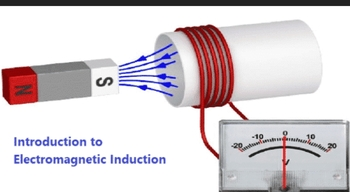Application of Electromagnetic InductionApplications of Electromagnetic Induction

Based on his experiments we now have Faraday’s law according to which the amount of voltage induced in a coil is proportional to the number of turns of the coil and the rate of changing magnetic field.

• AC generator works on the principle of electromagnetic induction.
• The working of electrical transformers are based on the electromagnetic induction.
• The magnetic flow meter is based on the electromagnetic induction.

Electromagnetic Induction Formula

Mathematically, the induced voltage can be given by the following relation:

 e = N × dΦ / dt

Where,

• e is the induced voltage (in volts)
• N is the number of turns in the coil
• Φ is the magnetic flux – the amount of magnetic field at a surface (in Webbers)
• t is the time (in seconds)

The significance of this discovery is a way of producing electrical energy in a circuit by using magnetic fields and not just batteries. Everyday machines like motors, generators and transformers work on the principle of electromagnetic induction.